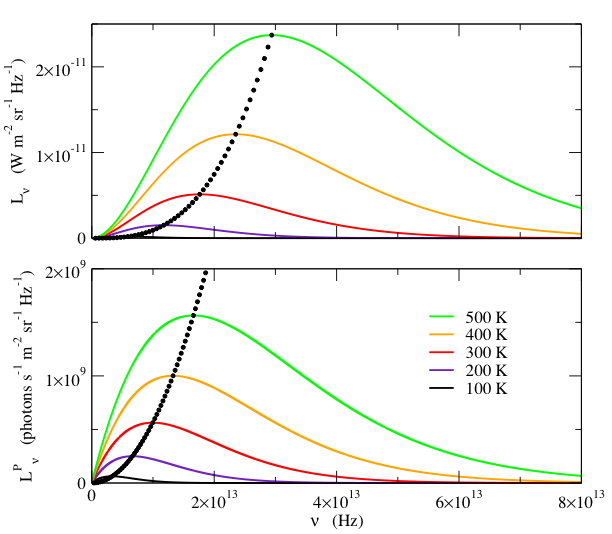Blackbody Calculator

The Planck Blackbody Formula in Units of Frequency

(click on equations to view enlarged)

It can be shown that the power emitted per unit projected area of a blackbody at temperature T, into a unit solid angle, in frequency interval ν to ν +dν, is(1)where h is Planck’s constant (6.6260693×1034   W s2) ,

c is the speed of light (2.99792458×108  m s-1)  and

k is Boltzmann’s constant (1.380658×1023  J K-1) .

This is the Planck blackbody formula (in one of many forms).  The quantity Lν is referred to as the spectral radiance.  The frequency of the maximum spectral radiance is found by setting the derivative with respect to ν equal to zero:This gives the transcendental equation  3(1 - e -x) = x,  where x = hν/kT .  Evaluating this numerically yields x = a3 ≈ 2.82143937212, so.                                                 (2)(We use the subscript 3 to refer to the coefficient in the transcendental equation, other versions of which we will encounter).  Substituting this in (1) gives(3)Many devices and systems respond in proportion to the number of incident photons, and it is useful to express radiometric quantities in terms of photons per second rather than watts.  Dividing the spectral radiance Lν (Eq. 1) by the energy of a photon, hν, gives the spectral photon radiance.                           (4)The peak of LνP occurs whenThis gives the transcendental equation 2(1 - e-x) = x, where x = hν/kT, with solution x = a2 ≈ 1.59362426004.  The peak spectral photon radiance thus occurs at frequency.                                                 (5)Note that the peak of LνP occurs at a lower frequency than Lν.  The peak value of LνP is found by substituting (5) into (4):(6)Both Lν and LνP are shown in Fig. 1, for several temperatures.Fig. 1-Spectral radiance, Lν, (top) and the spectral photon radiance, LνP, (bottom) as a function of frequency, ν, for various temperatures.  The small black dots indicate the frequency and value of the peak, at 10 K temperature intervals.  Note that Lν and LνP have different frequency dependences.  Although the peak frequency is proportional to T for both quantities, Lν peaks at a higher frequency than LνP.  Furthermore, the peak value of Lν increases as 3, whereas the peak value of LνP increases as 2.c.f . “Radiometry and the Detection of Optical Radiation,” by Robert W. Boyd, Wiley and Sons, 1983

 Calculation of a Blackbody Radiance Units of Frequency Units of Wavelength Units of Wavenumbers Radiance: Integrating the Planck Equation In-band Radiance: Integrating the Planck Equation over a Finite Range Appendix A: Algorithms for Computing In-band Radiance Appendix B: The Doppler Effect Appendix C: Summary of Formulas References Blackbody Calculator Print Version